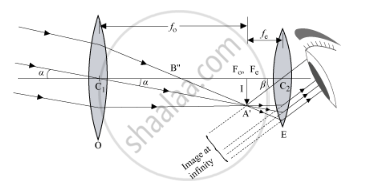# Draw a Labeled Ray Diagram to Obtain the Real Image Formed by an Astronomical Telescope in Normal Adjustment Position. Define Its Magnifying Power - Physics

Draw a labeled ray diagram to obtain the real image formed by an astronomical telescope in normal adjustment position. Define its magnifying power.

#### Solution

In normal adjustment, the final image is formed at infinity.Magnifying power or angular magnification of astronomical telescope: It is defined as the ratio of the angle subtended at the eye by the final image to the angle subtended at the eye, by the object directly, when the final image and the object, both are at infinity.

Angular magnification,M=beta/alpha

β and α are very small.

:.beta~~tan beta

alpha~~tanalpha

=>M=tanbeta/tanalpha

I is the image formed by the objective. f0 and fe are the focal lengths of the objective and eyepiece, respectively.

Here,

tanalpha=I/f_0

tan beta=I/-f_e

(Distance of the image from the eyepiece is taken as negative.)

:.M=((-I)/f_e)/(I/f_0)

 M=(-f_0)/f_e

Concept: Optical Instruments - Telescope
Is there an error in this question or solution?
2015-2016 (March) All India Set 2 C

Share